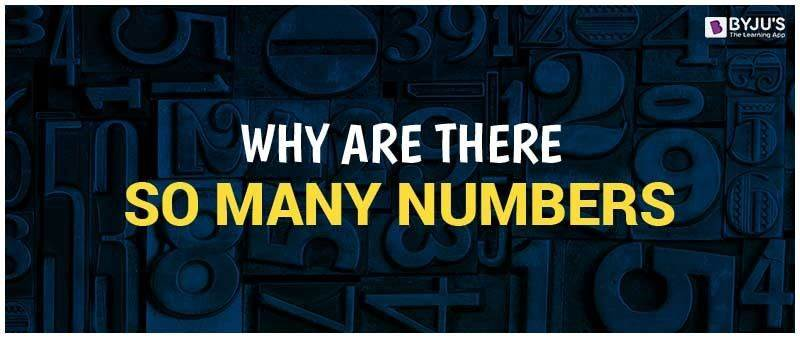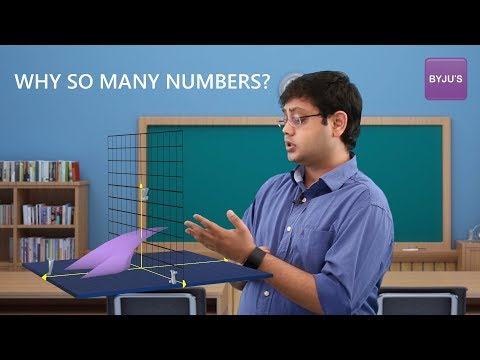# Why Are There So Many Numbers?As homo sapiens started to develop their cognitive ability, there came a need for a system to count, label and measure items. The first examples wherein a number system was found was a really long long time ago, wherein bones were found with tally marks showing possible marking of days or for counting sheep. But as the tally system lacked a place value, the system was quite impractical. Then came in a different number system with like the Mesopotamian base 60 system in BC 3400 and the base 10 system from Egypt in 3100 BC.

The next drastic change came by the use of 0 by Brahmagupta in AD 628. Zero helped to improve the base 10 system by enabling better place value better, saving space and signify null quantities.

But as the way of life continued to develop, the growing civilization brought in the concept of money to settle accounts. With money came debts which brought the need for a more appropriate system incorporating a broader spectrum of applications. This is wherein negative numbers came into the picture. Thus, positive and negative numbers formed the integers.

This was again not enough, as regions were being conquered and divided, then came the need for fractions. But as we dug deeper and deeper in our quest for knowledge, then we brought rational and irrational numbers into the system.

The name rational coming from fractions as they are in fact ratios of numbers. But then certain philosophers and scholars found patterns in the world we live in and postulated new theorems which further improved our knowledge of the system. Like how the Pythagorean theorem helped to find the place of √2 on a number scale. As the law proved there is no exact point to be marked, irrational numbers were the name given to them.

Then came a matter of representation on a 2-D plane. For this, topics like algebra and geometry were joined to create a 2-dimensional system, the Cartesian coordinate system. This system is used to map numbers according to algebraic expressions. But as equations started to get complex, so did the coordinate system.

Then again while solving algebraic expressions, another complication occurred. This was to find the root of a negative number. Since it’s not a domain that was known then, a third axis was added to compensate for the imaginary plain. Euler even assigned the number √1 with a symbol “i”. This brought forward a set of numbers which are called as the imaginary numbers. But visualizing the concept of a third axis is difficult on paper but easier to understand in a 3-D representation, say through animations.

Thus, numbers are a superset of many subsets. The subsets being, natural numbers, whole numbers, integers, rational numbers, real number and complex numbers.

Still confused about the number system, check out this video with the proper images and animation to help you understand the number system better.

##### Why are there so many numbers?To watch more fun facts and tips, register with BYJU’s and check out our Youtube channel.

 NCERT Related Articles NCERT Solutions for Class 12 NCERT Solutions for Class 11 NCERT Solutions for Class 10 NCERT Solutions for Class 12 Maths NCERT Solutions for Class 11 Maths NCERT Solutions for Class 10 Maths NCERT Solutions for Class 12 Physics NCERT Solutions for Class 11 Physics NCERT Solutions for Class 10 Science NCERT Solutions for Class 12 Chemistry NCERT Solutions for Class 11 Chemistry NCERT Solutions for Class 6 NCERT Solutions for Class 12 Biology NCERT Solutions for Class 11 Biology NCERT Solutions for Class 6 Maths NCERT Solutions for Class 9 NCERT Solutions for Class 8 NCERT Solutions for Class 6 Science NCERT Solutions for Class 9 Maths NCERT Solutions for Class 8 Maths NCERT Solutions for Class 4 NCERT Solutions for Class 9 Science NCERT Solutions for Class 8 Science NCERT Solutions for Class 4 Maths NCERT Solutions for Class 5 NCERT Solutions for Class 7 NCERT Solutions for Class 7 Maths NCERT Solutions for Class 4 Science NCERT Solutions for Class 5 Maths NCERT Solutions for Class 7 Science NCERT Solutions NCERT Books NCERT Solutions for Class 5 Science